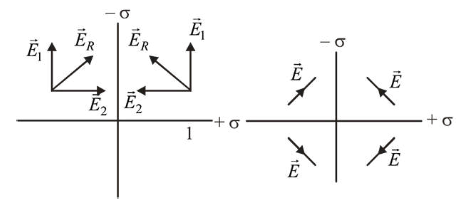# Two charged thin infinite plane sheets of uniform surface charge densityQuestion:

Two charged thin infinite plane sheets of uniform surface charge density $\sigma_{+}$and $\sigma_{-}$, where $\left|\sigma_{+}\right|>\left|\sigma_{-}\right|$, intersect at right angle. Which of the following best represents the electric field lines for this system ?

1.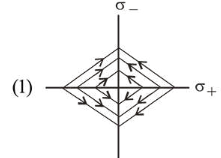2.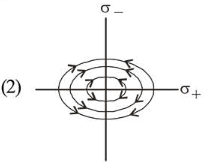3.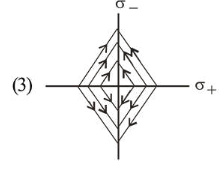4.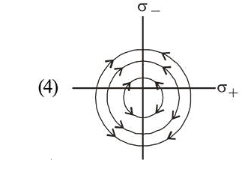Correct Option: , 3

Solution:

(3) The electric field produced due to uniformly charged infinite plane is uniform. So option (2) and (4) are wrong.

And +ve charge density $\sigma_{+}$is bigger in magnitude so its field along $Y$ direction will be bigger than field of-ve charge density $\sigma_{-}$in $X$ direction. Hence option (3) is correct.